Anzeige

# Lecture notes 02

27. Jun 2011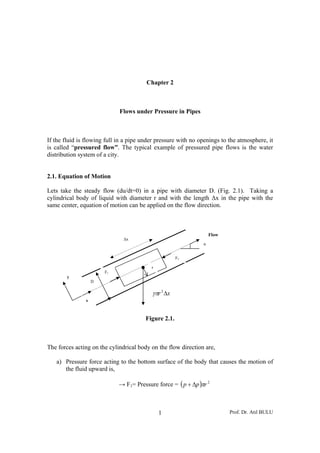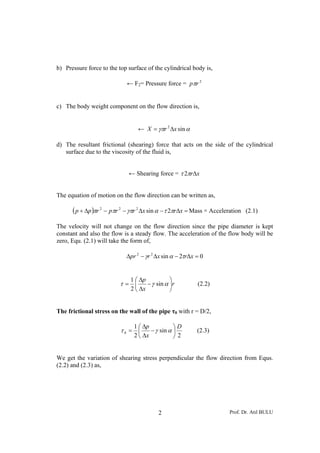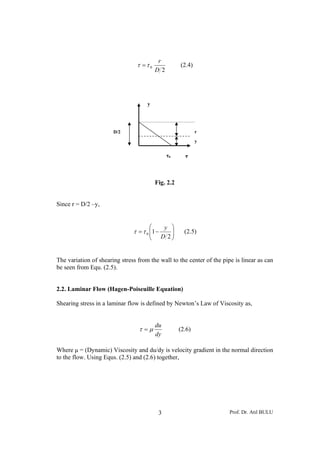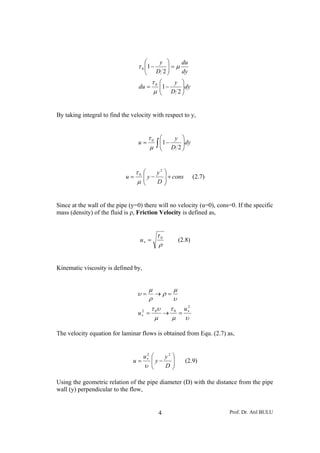Anzeige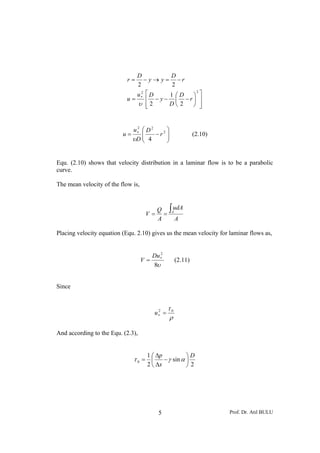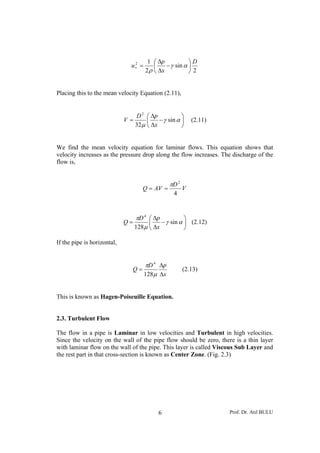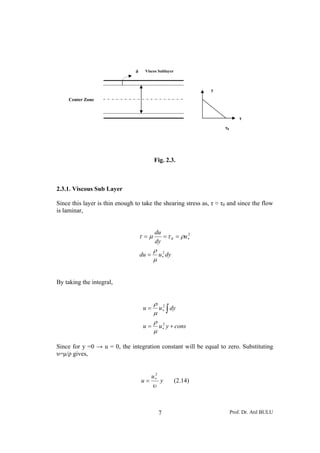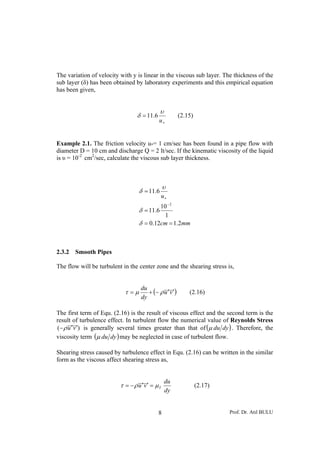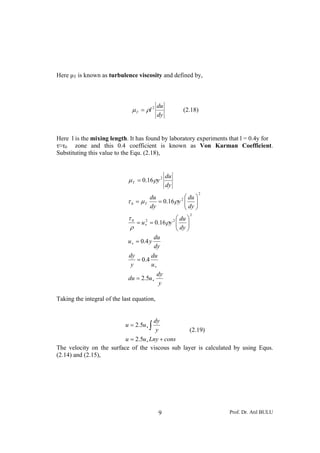Anzeige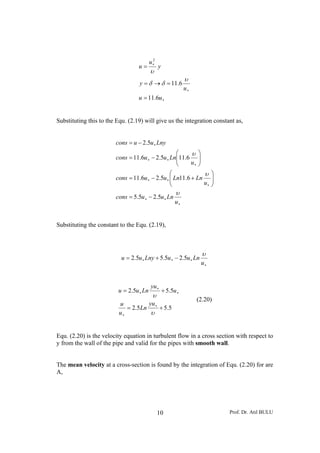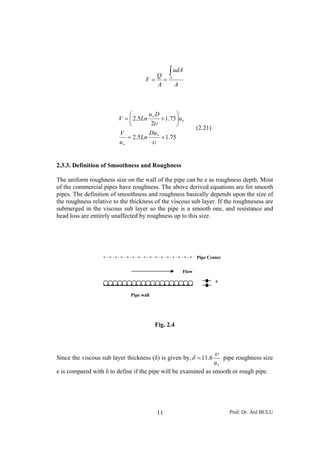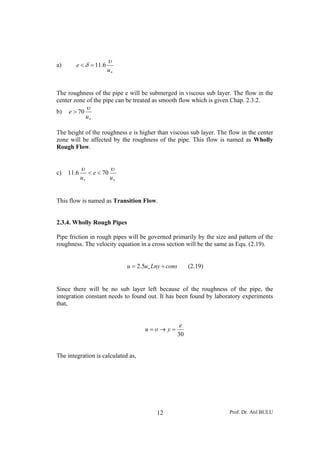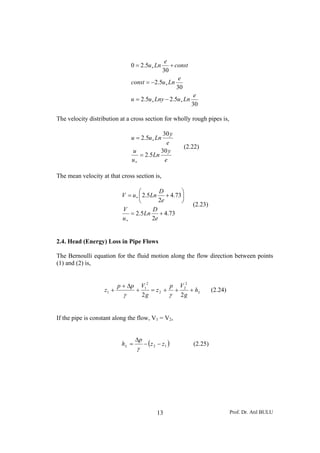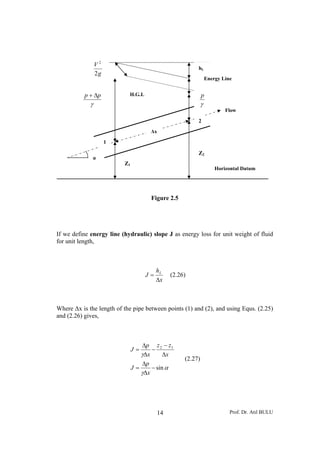Anzeige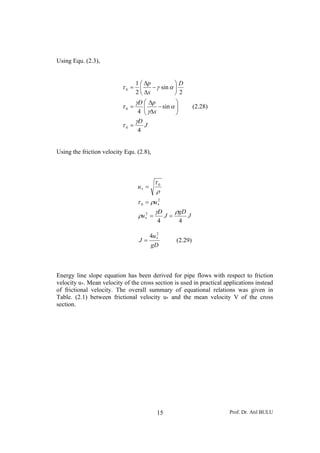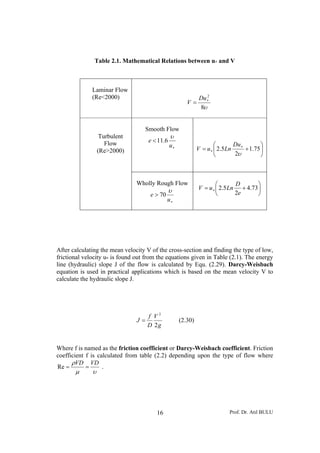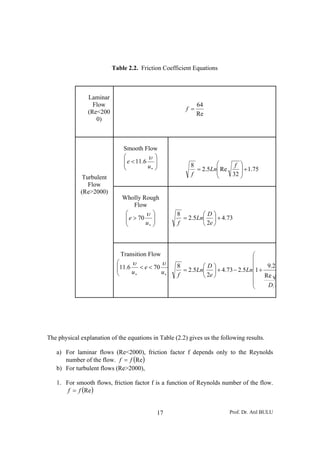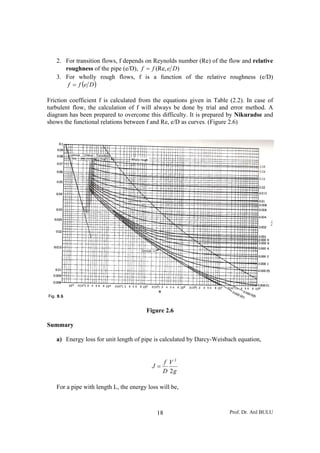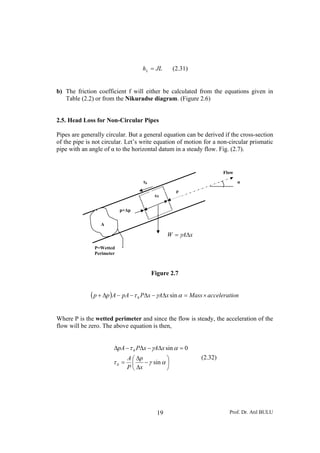Anzeige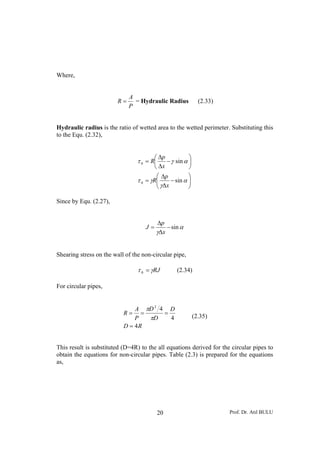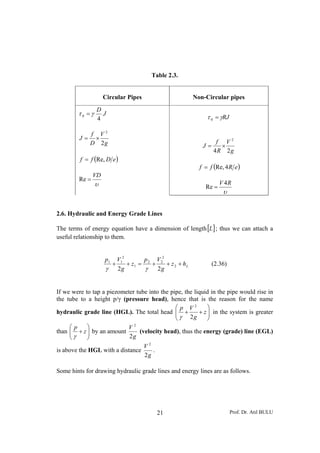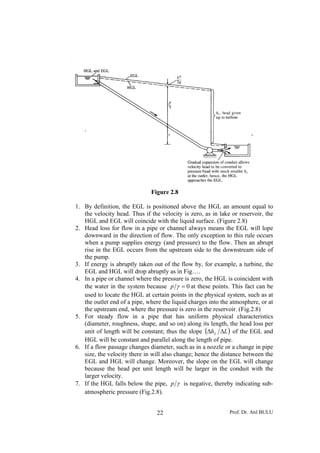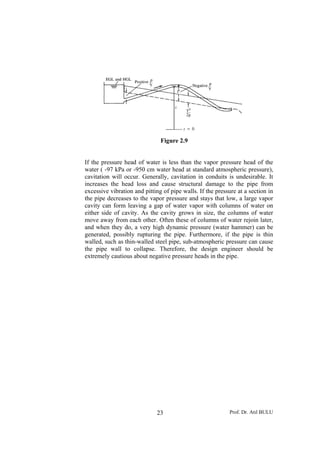Nächste SlideShare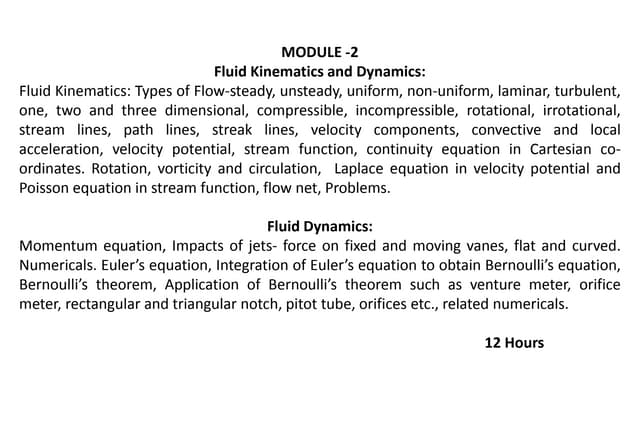Fluid kinematics
1 von 24
Anzeige

### Lecture notes 02

1. Chapter 2 Flows under Pressure in Pipes If the fluid is flowing full in a pipe under pressure with no openings to the atmosphere, it is called “pressured flow”. The typical example of pressured pipe flows is the water distribution system of a city. 2.1. Equation of Motion Lets take the steady flow (du/dt=0) in a pipe with diameter D. (Fig. 2.1). Taking a cylindrical body of liquid with diameter r and with the length Δx in the pipe with the same center, equation of motion can be applied on the flow direction. Flow Δx α F2 r F1 y D γπr 2 Δx x Figure 2.1. The forces acting on the cylindrical body on the flow direction are, a) Pressure force acting to the bottom surface of the body that causes the motion of the fluid upward is, → F1= Pressure force = ( p + Δp )πr 2 1 Prof. Dr. Atıl BULU
2. b) Pressure force to the top surface of the cylindrical body is, ← F2= Pressure force = pπr 2 c) The body weight component on the flow direction is, ← X = γπr 2 Δx sin α d) The resultant frictional (shearing) force that acts on the side of the cylindrical surface due to the viscosity of the fluid is, ← Shearing force = τ 2πrΔx The equation of motion on the flow direction can be written as, ( p + Δp )πr 2 − pπr 2 − γπr 2 Δx sin α − τ 2πrΔx = Mass × Acceleration (2.1) The velocity will not change on the flow direction since the pipe diameter is kept constant and also the flow is a steady flow. The acceleration of the flow body will be zero, Equ. (2.1) will take the form of, Δpr 2 − γr 2 Δx sin α − 2τrΔx = 0 1 ⎛ Δp ⎞ τ= ⎜ − γ sin α ⎟r (2.2) 2 ⎝ Δx ⎠ The frictional stress on the wall of the pipe τ0 with r = D/2, 1 ⎛ Δp ⎞D τ0 = ⎜ − γ sin α ⎟ (2.3) 2 ⎝ Δx ⎠2 We get the variation of shearing stress perpendicular the flow direction from Equs. (2.2) and (2.3) as, 2 Prof. Dr. Atıl BULU
3. r τ =τ0 (2.4) D2 y D/2 r y τ0 τ Fig. 2.2 Since r = D/2 –y, ⎛ y ⎞ τ = τ 0 ⎜1 − ⎜ ⎟ (2.5) ⎝ D 2⎟ ⎠ The variation of shearing stress from the wall to the center of the pipe is linear as can be seen from Equ. (2.5). 2.2. Laminar Flow (Hagen-Poiseuille Equation) Shearing stress in a laminar flow is defined by Newton’s Law of Viscosity as, du τ =μ (2.6) dy Where μ = (Dynamic) Viscosity and du/dy is velocity gradient in the normal direction to the flow. Using Equs. (2.5) and (2.6) together, 3 Prof. Dr. Atıl BULU
4. y ⎞ du τ 0 ⎜1 − ⎜ ⎟=μ ⎟ ⎝ D 2⎠ dy τ0 ⎛ y ⎞ du = ⎜1 − ⎜ D 2 ⎟dy ⎟ μ ⎝ ⎠ By taking integral to find the velocity with respect to y, τ0 ⎛ y ⎞ u= μ ∫ ⎜1 − D 2 ⎟dy ⎜ ⎝ ⎟ ⎠ τ0 ⎛ y2 ⎞ u= ⎜y− ⎜ ⎟ + cons (2.7) μ ⎝ D⎟ ⎠ Since at the wall of the pipe (y=0) there will no velocity (u=0), cons=0. If the specific mass (density) of the fluid is ρ, Friction Velocity is defined as, τ0 u∗ = (2.8) ρ Kinematic viscosity is defined by, μ μ υ= →ρ= ρ υ τ 0υ τ 0 u ∗2 u∗ = 2 → = μ μ υ The velocity equation for laminar flows is obtained from Equ. (2.7) as, u∗ ⎛ 2 y2 ⎞ u= ⎜y− ⎟ (2.9) υ ⎜⎝ D⎟ ⎠ Using the geometric relation of the pipe diameter (D) with the distance from the pipe wall (y) perpendicular to the flow, 4 Prof. Dr. Atıl BULU
5. D D r= − y → y = −r 2 2 2 ⎡ ⎞ ⎤ 2 u D 1 ⎛D u = ∗ ⎢ − y − ⎜ −r⎟ ⎥ υ ⎢2 ⎣ D⎝ 2 ⎠ ⎥⎦ u∗ ⎛ D 2 2 2 ⎞ u= ⎜ ⎜ 4 −r ⎟⎟ (2.10) υD ⎝ ⎠ Equ. (2.10) shows that velocity distribution in a laminar flow is to be a parabolic curve. The mean velocity of the flow is, Q ∫AudA V= = A A Placing velocity equation (Equ. 2.10) gives us the mean velocity for laminar flows as, 2 Du ∗ V= (2.11) 8υ Since τ0 u∗ = 2 ρ And according to the Equ. (2.3), 1 ⎛ Δp ⎞D τ0 = ⎜ − γ sin α ⎟ 2 ⎝ Δx ⎠2 5 Prof. Dr. Atıl BULU
6. 1 ⎛ Δp ⎞D u∗ = 2 ⎜ − γ sin α ⎟ 2 ρ ⎝ Δx ⎠2 Placing this to the mean velocity Equation (2.11), D 2 ⎛ Δp ⎞ V= ⎜ − γ sin α ⎟ (2.11) 32μ ⎝ Δx ⎠ We find the mean velocity equation for laminar flows. This equation shows that velocity increases as the pressure drop along the flow increases. The discharge of the flow is, πD 2 Q = AV = V 4 πD 4 ⎛ Δp ⎞ Q= ⎜ − γ sin α ⎟ (2.12) 128μ ⎝ Δx ⎠ If the pipe is horizontal, πD 4 Δp Q= (2.13) 128μ Δx This is known as Hagen-Poiseuille Equation. 2.3. Turbulent Flow The flow in a pipe is Laminar in low velocities and Turbulent in high velocities. Since the velocity on the wall of the pipe flow should be zero, there is a thin layer with laminar flow on the wall of the pipe. This layer is called Viscous Sub Layer and the rest part in that cross-section is known as Center Zone. (Fig. 2.3) 6 Prof. Dr. Atıl BULU
7. δ Viscos Sublayer y Center Zone τ τ0 Fig. 2.3. 2.3.1. Viscous Sub Layer Since this layer is thin enough to take the shearing stress as, τ ≈ τ0 and since the flow is laminar, du τ =μ = τ 0 = ρu ∗ 2 dy ρ 2 du = u ∗ dy μ By taking the integral, ρ 2 μ ∫ u= u ∗ dy ρ 2 u = u ∗ y + cons μ Since for y =0 → u = 0, the integration constant will be equal to zero. Substituting υ=μ/ρ gives, 2 u∗ u= y (2.14) υ 7 Prof. Dr. Atıl BULU
8. The variation of velocity with y is linear in the viscous sub layer. The thickness of the sub layer (δ) has been obtained by laboratory experiments and this empirical equation has been given, υ δ = 11.6 (2.15) u∗ Example 2.1. The friction velocity u*= 1 cm/sec has been found in a pipe flow with diameter D = 10 cm and discharge Q = 2 lt/sec. If the kinematic viscosity of the liquid is υ = 10-2 cm2/sec, calculate the viscous sub layer thickness. υ δ = 11.6 u∗ 10 − 2 δ = 11.6 1 δ = 0.12cm = 1.2mm 2.3.2 Smooth Pipes The flow will be turbulent in the center zone and the shearing stress is, + (− ρ u ′ v ′) du τ =μ (2.16) dy The first term of Equ. (2.16) is the result of viscous effect and the second term is the result of turbulence effect. In turbulent flow the numerical value of Reynolds Stress (− ρ u ′ v ′) is generally several times greater than that of (μ du dy ) . Therefore, the viscosity term (μ du dy ) may be neglected in case of turbulent flow. Shearing stress caused by turbulence effect in Equ. (2.16) can be written in the similar form as the viscous affect shearing stress as, du τ = −ρ u ′v ′ = μ T (2.17) dy 8 Prof. Dr. Atıl BULU
9. Here μT is known as turbulence viscosity and defined by, du μ T = ρl 2 (2.18) dy Here l is the mixing length. It has found by laboratory experiments that l = 0.4y for τ≈τ0 zone and this 0.4 coefficient is known as Von Karman Coefficient. Substituting this value to the Equ. (2.18), du μ T = 0.16 ρy 2 dy 2 du ⎛ du ⎞ τ 0 = μT = 0.16 ρy 2 ⎜ ⎟ ⎜ dy ⎟ dy ⎝ ⎠ 2 τ0 ⎛ du ⎞ = u ∗ = 0.16 ρy 2 ⎜ ⎟ 2 ⎜ dy ⎟ ρ ⎝ ⎠ du u ∗ = 0.4 y dy dy du = 0.4 y u∗ dy du = 2.5u ∗ y Taking the integral of the last equation, dy u = 2.5u ∗ ∫ y (2.19) u = 2.5u ∗ Lny + cons The velocity on the surface of the viscous sub layer is calculated by using Equs. (2.14) and (2.15), 9 Prof. Dr. Atıl BULU
10. 2 u∗ u= y υ υ y = δ → δ = 11.6 u∗ u = 11.6u ∗ Substituting this to the Equ. (2.19) will give us the integration constant as, cons = u − 2.5u ∗ Lny ⎛ υ ⎞ cons = 11.6u ∗ − 2.5u ∗ Ln⎜11.6 ⎜ ⎟ ⎟ ⎝ u∗ ⎠ ⎛ υ ⎞ cons = 11.6u ∗ − 2.5u ∗ ⎜ Ln11.6 + Ln ⎜ ⎟ ⎟ ⎝ u∗ ⎠ υ cons = 5.5u ∗ − 2.5u ∗ Ln u∗ Substituting the constant to the Equ. (2.19), υ u = 2.5u ∗ Lny + 5.5u ∗ − 2.5u ∗ Ln u∗ yu ∗ u = 2.5u ∗ Ln + 5.5u ∗ υ (2.20) u yu = 2.5 Ln ∗ + 5.5 u∗ υ Equ. (2.20) is the velocity equation in turbulent flow in a cross section with respect to y from the wall of the pipe and valid for the pipes with smooth wall. The mean velocity at a cross-section is found by the integration of Equ. (2.20) for are A, 10 Prof. Dr. Atıl BULU
11. Q ∫ udA V= = A A A ⎛ u D ⎞ V = ⎜ 2.5Ln ∗ + 1.75 ⎟u ∗ ⎝ 2υ ⎠ (2.21) V Du ∗ = 2.5 Ln + 1.75 u∗ υ 2.3.3. Definition of Smoothness and Roughness The uniform roughness size on the wall of the pipe can be e as roughness depth. Most of the commercial pipes have roughness. The above derived equations are for smooth pipes. The definition of smoothness and roughness basically depends upon the size of the roughness relative to the thickness of the viscous sub layer. If the roughnesess are submerged in the viscous sub layer so the pipe is a smooth one, and resistance and head loss are entirely unaffected by roughness up to this size. Pipe Center Flow e Pipe wall Fig. 2.4 υ Since the viscous sub layer thickness (δ) is given by, δ = 11.6 pipe roughness size u∗ e is compared with δ to define if the pipe will be examined as smooth or rough pipe. 11 Prof. Dr. Atıl BULU
12. υ a) e < δ = 11.6 u∗ The roughness of the pipe e will be submerged in viscous sub layer. The flow in the center zone of the pipe can be treated as smooth flow which is given Chap. 2.3.2. υ b) e > 70 u∗ The height of the roughness e is higher than viscous sub layer. The flow in the center zone will be affected by the roughness of the pipe. This flow is named as Wholly Rough Flow. υ υ c) 11.6 < e < 70 u∗ u∗ This flow is named as Transition Flow. 2.3.4. Wholly Rough Pipes Pipe friction in rough pipes will be governed primarily by the size and pattern of the roughness. The velocity equation in a cross section will be the same as Equ. (2.19). u = 2.5u ∗ Lny + cons (2.19) Since there will be no sub layer left because of the roughness of the pipe, the integration constant needs to found out. It has been found by laboratory experiments that, e u=o→ y= 30 The integration is calculated as, 12 Prof. Dr. Atıl BULU
13. e 0 = 2.5u ∗ Ln + const 30 e const = −2.5u ∗ Ln 30 e u = 2.5u ∗ Lny − 2.5u ∗ Ln 30 The velocity distribution at a cross section for wholly rough pipes is, 30 y u = 2.5u ∗ Ln e (2.22) u 30 y = 2.5Ln u∗ e The mean velocity at that cross section is, ⎛ D ⎞ V = u ∗ ⎜ 2.5Ln + 4.73 ⎟ ⎝ 2e ⎠ (2.23) V D = 2.5Ln + 4.73 u∗ 2e 2.4. Head (Energy) Loss in Pipe Flows The Bernoulli equation for the fluid motion along the flow direction between points (1) and (2) is, p + Δp V12 p V2 z1 + + = z 2 + + 2 + hL (2.24) γ 2g γ 2g If the pipe is constant along the flow, V1 = V2, Δp hL = − ( z 2 − z1 ) (2.25) γ 13 Prof. Dr. Atıl BULU
14. V2 hL 2g Energy Line p + Δp H.G.L p γ γ Flow 2 Δx 1 Z2 α Z1 Horizontal Datum Figure 2.5 If we define energy line (hydraulic) slope J as energy loss for unit weight of fluid for unit length, hL J= (2.26) Δx Where Δx is the length of the pipe between points (1) and (2), and using Equs. (2.25) and (2.26) gives, Δp z 2 − z 1 J= − γΔx Δx (2.27) Δp J= − sin α γΔx 14 Prof. Dr. Atıl BULU
15. Using Equ. (2.3), 1 ⎛ Δp ⎞D τ0 = ⎜ − γ sin α ⎟ 2 ⎝ Δx ⎠2 γD ⎛ Δp ⎞ τ0 = ⎜ γΔx − sin α ⎟ ⎜ ⎟ (2.28) 4 ⎝ ⎠ γD τ0 = J 4 Using the friction velocity Equ. (2.8), τ0 u∗ = ρ τ 0 = ρu ∗2 γD ρgD ρu ∗2 = J= J 4 4 2 4u ∗ J= (2.29) gD Energy line slope equation has been derived for pipe flows with respect to friction velocity u*. Mean velocity of the cross section is used in practical applications instead of frictional velocity. The overall summary of equational relations was given in Table. (2.1) between frictional velocity u* and the mean velocity V of the cross section. 15 Prof. Dr. Atıl BULU
16. Table 2.1. Mathematical Relations between u* and V Laminar Flow (Re<2000) 2 Du ∗ V= 8υ Smooth Flow Turbulent υ Flow e < 11.6 u∗ ⎛ Du ∗ ⎞ (Re>2000) V = u ∗ ⎜ 2.5Ln + 1.75 ⎟ ⎝ 2υ ⎠ Wholly Rough Flow ⎛ D ⎞ υ V = u ∗ ⎜ 2.5 Ln + 4.73 ⎟ e > 70 ⎝ 2e ⎠ u∗ After calculating the mean velocity V of the cross-section and finding the type of low, frictional velocity u* is found out from the equations given in Table (2.1). The energy line (hydraulic) slope J of the flow is calculated by Equ. (2.29). Darcy-Weisbach equation is used in practical applications which is based on the mean velocity V to calculate the hydraulic slope J. f V2 J= (2.30) D 2g Where f is named as the friction coefficient or Darcy-Weisbach coefficient. Friction coefficient f is calculated from table (2.2) depending upon the type of flow where ρVD VD Re = = . μ υ 16 Prof. Dr. Atıl BULU
17. Table 2.2. Friction Coefficient Equations Laminar Flow 64 (Re<200 f = Re 0) Smooth Flow ⎛ υ ⎞ ⎜ e < 11.6 ⎟ ⎜ ⎝ u∗ ⎟ ⎠ 8 ⎛ = 2.5Ln⎜ Re f ⎞ ⎟ + 1.75 f ⎜ 32 ⎟ Turbulent ⎝ ⎠ Flow (Re>2000) Wholly Rough Flow ⎛ υ ⎞ 8 ⎛D⎞ ⎜ e > 70 ⎟ ⎜ = 2.5Ln⎜ ⎟ + 4.73 ⎝ u∗ ⎟ ⎠ f ⎝ 2e ⎠ Transition Flow ⎛ ⎜ ⎛ υ υ ⎜ ⎜11.6 < e < 70 8 ⎛D⎞ 9.2 ⎜ = 2.5Ln⎜ ⎟ + 4.73 − 2.5Ln⎜1 + ⎝ u∗ u∗ ⎝ 2e ⎠ f ⎜ Re ⎜ ⎝ De The physical explanation of the equations in Table (2.2) gives us the following results. a) For laminar flows (Re<2000), friction factor f depends only to the Reynolds number of the flow. f = f (Re ) b) For turbulent flows (Re>2000), 1. For smooth flows, friction factor f is a function of Reynolds number of the flow. f = f (Re ) 17 Prof. Dr. Atıl BULU
18. 2. For trannsition flow f depend on Reyno number (Re) of the flow and relative ws, ds olds r e r roughn of the pipe (e/D), f = f (Re, e D) ness p 3. For wh holly rough flows, f is a fun h nction of t the relative roughness (e/D) e f = f (e D ) Fric ction coeffic cient f is c calculated fr from the eq quations giv in Tabl (2.2). In case of ven le turb bulent flow, the calcul , lation of f will always be done by trial and error method. A diag gram has be prepare to overco een ed fficulty. It i prepared by Nikura ome this dif is adse and show the func ws ctional relati ions betwee f and Re, e/D as curv (Figure 2.6) en , ves. e Figure 2.6 Sum mmary a) Energy loss for uni length of pipe is calc it culated by D Darcy-Weisb bach equati ion, f V2 J= D 2g For a pipe w length L, the energy loss will be, with l 18 Prof. Dr. Atıl BULU A
19. h L = JL (2.31) b) The friction coefficient f will either be calculated from the equations given in Table (2.2) or from the Nikuradse diagram. (Figure 2.6) 2.5. Head Loss for Non-Circular Pipes Pipes are generally circular. But a general equation can be derived if the cross-section of the pipe is not circular. Let’s write equation of motion for a non-circular prismatic pipe with an angle of α to the horizontal datum in a steady flow. Fig. (2.7). Flow τ0 α p Δx p+Δp A W = γAΔx P=Wetted Perimeter Figure 2.7 ( p + Δp )A − pA − τ 0 PΔx − γAΔx sin α = Mass × acceleration Where P is the wetted perimeter and since the flow is steady, the acceleration of the flow will be zero. The above equation is then, ΔpA − τ 0 PΔx − γAΔx sin α = 0 A ⎛ Δp ⎞ (2.32) τ0 = ⎜ − γ sin α ⎟ P ⎝ Δx ⎠ 19 Prof. Dr. Atıl BULU
20. Where, A R= = Hydraulic Radius (2.33) P Hydraulic radius is the ratio of wetted area to the wetted perimeter. Substituting this to the Equ. (2.32), ⎛ Δp ⎞ τ 0 = R⎜ − γ sin α ⎟ ⎝ Δx ⎠ ⎛ Δp ⎞ τ 0 = γR⎜ ⎜ γΔx − sin α ⎟ ⎟ ⎝ ⎠ Since by Equ. (2.27), Δp J= − sin α γΔx Shearing stress on the wall of the non-circular pipe, τ 0 = γRJ (2.34) For circular pipes, A πD 2 4 D R= = = (2.35) P πD 4 D = 4R This result is substituted (D=4R) to the all equations derived for the circular pipes to obtain the equations for non-circular pipes. Table (2.3) is prepared for the equations as, 20 Prof. Dr. Atıl BULU
21. Table 2.3. Circular Pipes Non-Circular pipes D τ0 =γ J 4 τ 0 = γRJ f V2 J= × D 2g f V2 J= × 4R 2 g f = f (Re, D e ) f = f (Re, 4 R e ) VD Re = υ V 4R Re = υ 2.6. Hydraulic and Energy Grade Lines The terms of energy equation have a dimension of length [L ] ; thus we can attach a useful relationship to them. p1 V12 p V2 + + z1 = 2 + 2 + z 2 + h L (2.36) γ 2g γ 2g If we were to tap a piezometer tube into the pipe, the liquid in the pipe would rise in the tube to a height p/γ (pressure head), hence that is the reason for the name ⎛ p V2 ⎞ hydraulic grade line (HGL). The total head ⎜ + ⎜ γ 2g + z ⎟ in the system is greater ⎟ ⎝ ⎠ ⎛p ⎞ V2 than ⎜ + z ⎟ by an amount ⎜γ ⎟ (velocity head), thus the energy (grade) line (EGL) ⎝ ⎠ 2g V2 is above the HGL with a distance . 2g Some hints for drawing hydraulic grade lines and energy lines are as follows. 21 Prof. Dr. Atıl BULU
22. Figure 2.8 e 1. By definition, the EGL is positio oned above the HGL a amount equal to an e the velocity head. Thu if the vel y us locity is zer as in lak or reserv ro, ke voir, the HGL and E EGL will cooincide with the liquid s h surface. (Figgure 2.8) 2. Head loss f flow in a pipe or c for channel alw ways means the EGL will lope w downward in the direc ction of flow The only exception to this rule occurs w. y n e when a pum supplies energy (an pressure to the flo Then an abrupt mp s nd e) ow. n rise in the EGL occur from the upstream si to the d rs ide downstream side of m the pump. 3. If energy is abruptly t s taken out of the flow b for exam f by, mple, a turbbine, the EGL and H HGL will dro abruptly as in Fig… op y …. 4. In a pipe or channel w r where the pre essure is zer the HGL is coincide with ro, L ent the water in the system because p γ = 0 at these point This fact can be n m ts. t used to locate the HGL at certain points in th physical system, su as at L n he l uch the outlet e of a pipe where the liquid cha end e, e arges into th atmosphe or at he ere, the upstream end, whe the press m ere sure is zero in the reser rvoir. (Fig.2 2.8) 5. For steady flow in a pipe tha has unif y at form physi ical characcteristics (diameter, rroughness, shape, and so on) alon its length the head loss per ng h, unit of leng will be constant; th the slop (Δh L ΔL ) of the E gth hus pe EGL and HGL will b constant and parallel along the l be l length of pi ipe. 6. If a flow pa assage chan nges diamete such as i a nozzle or a change in pipe er, in e size, the ve elocity there in will also change; h e o hence the diistance betwween the EGL and H HGL will ch hange. Mor reover, the sslope on the EGL will change e l because the head per unit length will be la e h arger in the conduit with the e w larger veloccity. 7. If the HGL falls below the pipe, p γ is neg L w gative, there indicati sub- eby ing atmospheri pressure ( ic (Fig.2.8). 22 Prof. Dr. Atıl BULU A
23. Figure 2.9 If the pressure head of water is less than the vapor pressure head of the water ( -97 kPa or -950 cm water head at standard atmospheric pressure), cavitation will occur. Generally, cavitation in conduits is undesirable. It increases the head loss and cause structural damage to the pipe from excessive vibration and pitting of pipe walls. If the pressure at a section in the pipe decreases to the vapor pressure and stays that low, a large vapor cavity can form leaving a gap of water vapor with columns of water on either side of cavity. As the cavity grows in size, the columns of water move away from each other. Often these of columns of water rejoin later, and when they do, a very high dynamic pressure (water hammer) can be generated, possibly rupturing the pipe. Furthermore, if the pipe is thin walled, such as thin-walled steel pipe, sub-atmospheric pressure can cause the pipe wall to collapse. Therefore, the design engineer should be extremely cautious about negative pressure heads in the pipe. 23 Prof. Dr. Atıl BULU
24. 24 Prof. Dr. Atıl BULU
Anzeige